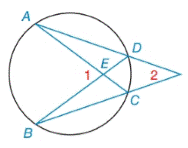Chapter 6.2, Problem 10E### Elementary Geometry for College St...

6th Edition
Daniel C. Alexander + 1 other
ISBN: 9781285195698

#### Solutions

Chapter
Section### Elementary Geometry for College St...

6th Edition
Daniel C. Alexander + 1 other
ISBN: 9781285195698
Textbook Problem
1 views

# Given: m ∠ 1=72 ° , m D C ⌢ = 34 ° Find: a) m A B ⌢ b) m ∠ 2To determine

(a)

To find:

mAB when m1=72° and mDC=34°.

Explanation

Theorem:

The measure of an angle formed by two chords that intersect that intersect within a circle is one-half the sum of the measure of the arcs intercepted by the angle and its vertical angle.

Calculation:

mAB when m1=72° and mDC=34°.

By Theorem,

m1=12(mAB+mDC)7

To determine

(b)

To find:

m2 when m1=72° and mDC=34°.

### Still sussing out bartleby?

Check out a sample textbook solution.

See a sample solution

#### The Solution to Your Study Problems

Bartleby provides explanations to thousands of textbook problems written by our experts, many with advanced degrees!

Get Started